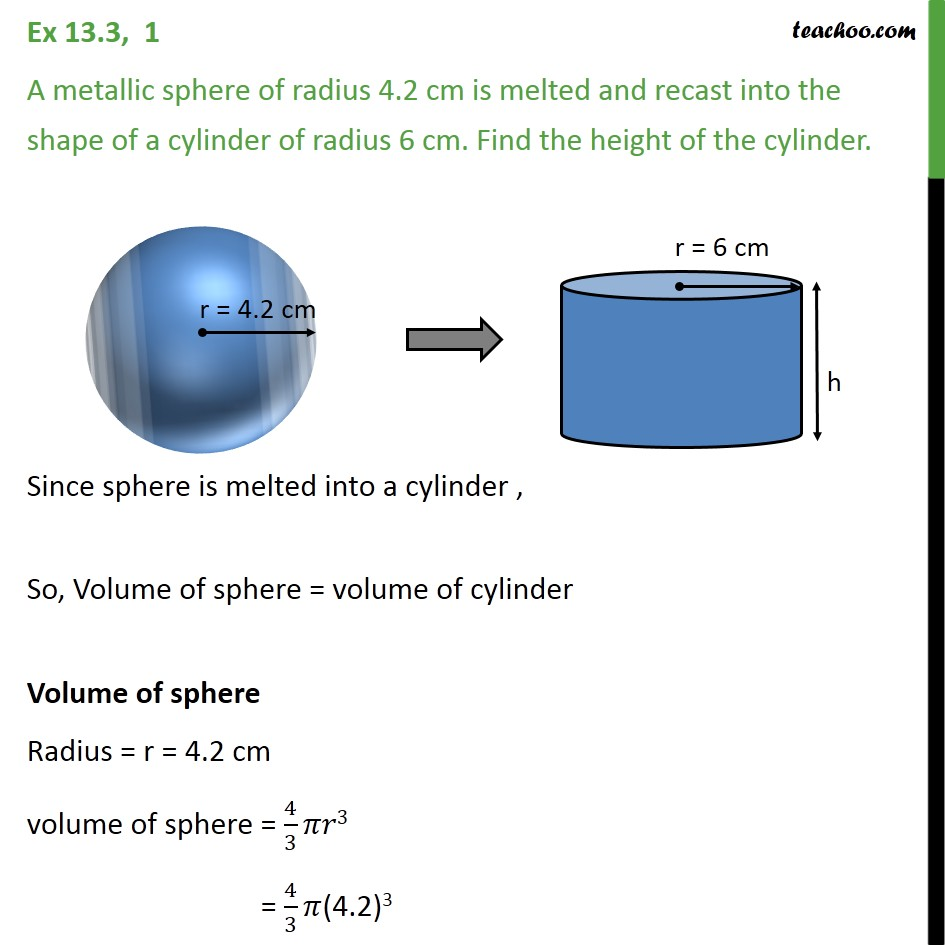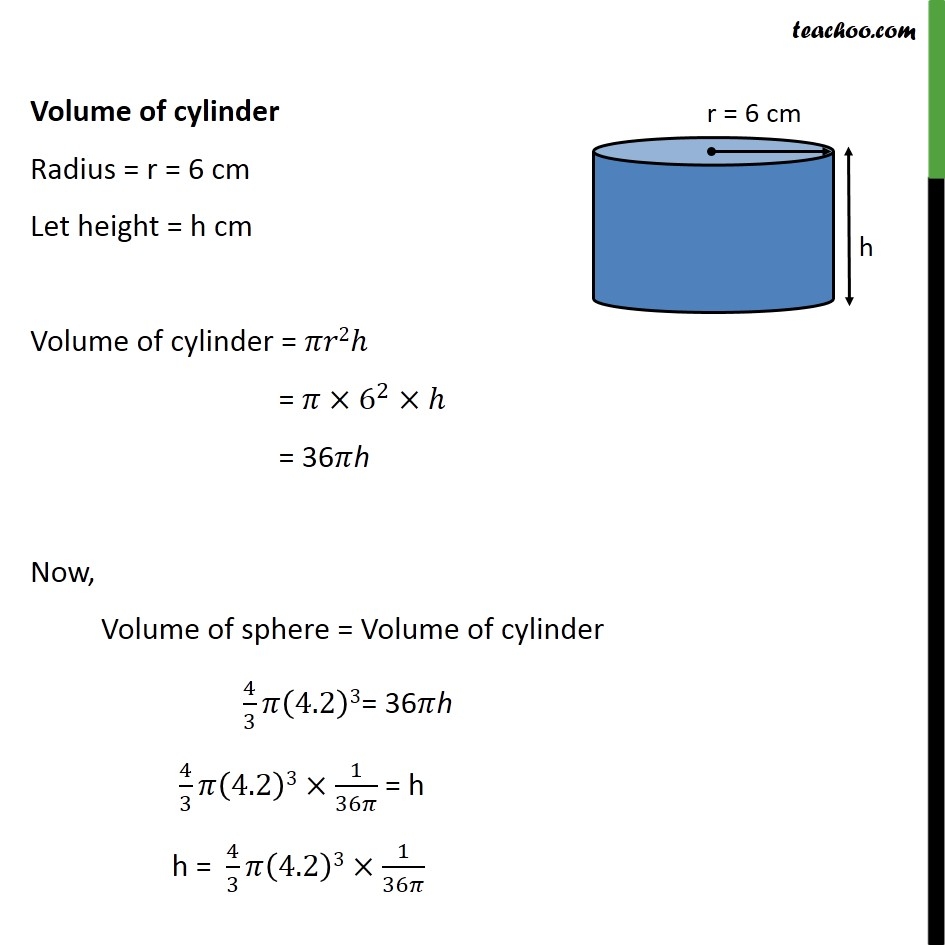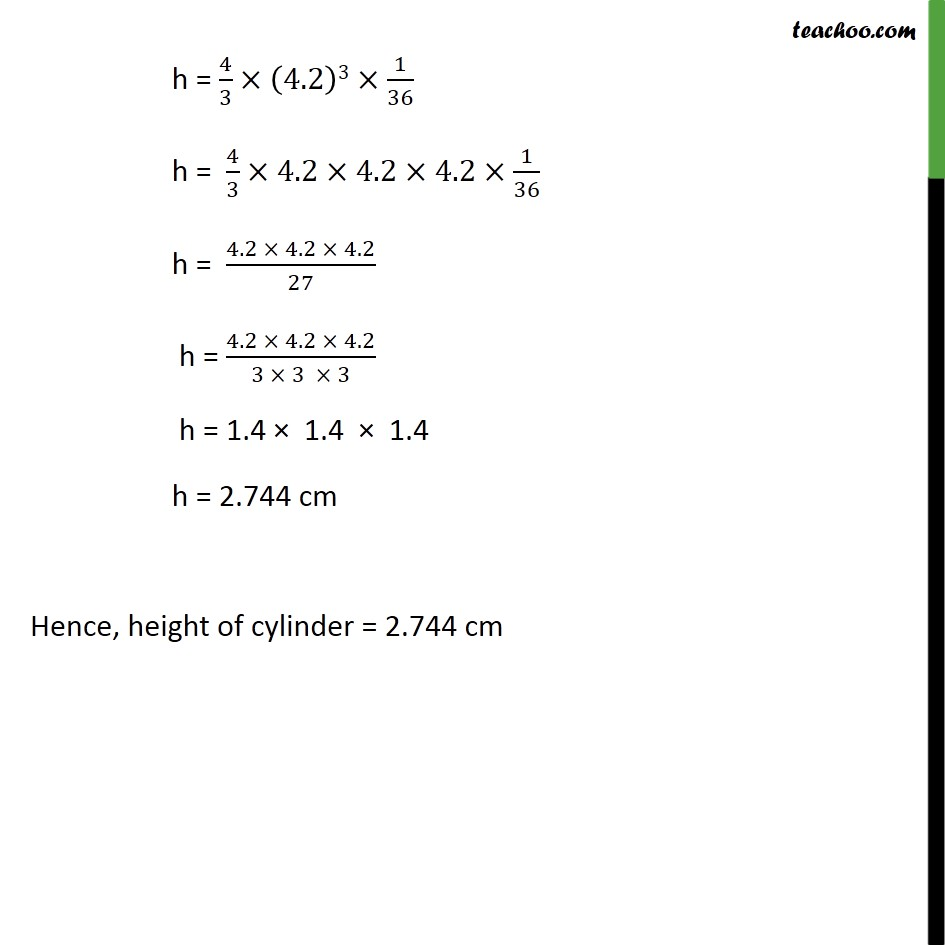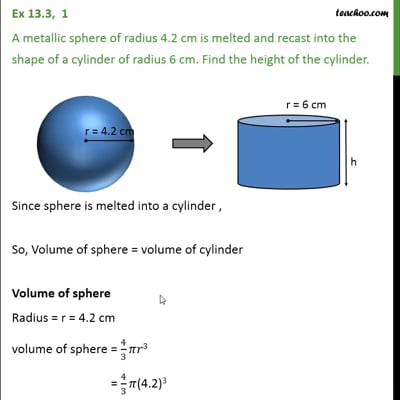Converting one shape to anotherThis video is only available for Teachoo black users

Learn in your speed, with individual attention - Teachoo Maths 1-on-1 Class

### Transcript

Question 1 A metallic sphere of radius 4.2 cm is melted and recast into the shape of a cylinder of radius 6 cm. Find the height of the cylinder. Since sphere is melted into a cylinder , So, Volume of sphere = volume of cylinder Volume of sphere Radius = r = 4.2 cm volume of sphere = 4/3 𝜋𝑟3 = 4/3 𝜋(4.2)3 Volume of cylinder Radius = r = 6 cm Let height = h cm Volume of cylinder = 𝜋𝑟2ℎ = 𝜋×6^2×ℎ = 36𝜋h Now, Volume of sphere = Volume of cylinder 4/3 𝜋(4.2)3= 36𝜋h 4/3 𝜋(4.2)3×1/36𝜋 = h h = 4/3 𝜋(4.2)3×1/36𝜋 h = 4/3×(4.2)3×1/36 h = 4/3×4.2×4.2×4.2×1/36 h = (4.2 × 4.2 × 4.2)/27 h = (4.2 × 4.2 × 4.2)/(3 × 3 × 3) h = 1.4 × 1.4 × 1.4 h = 2.744 cm Hence, height of cylinder = 2.744 cm